# Reordering

Strapi allows you to reorder a relation list.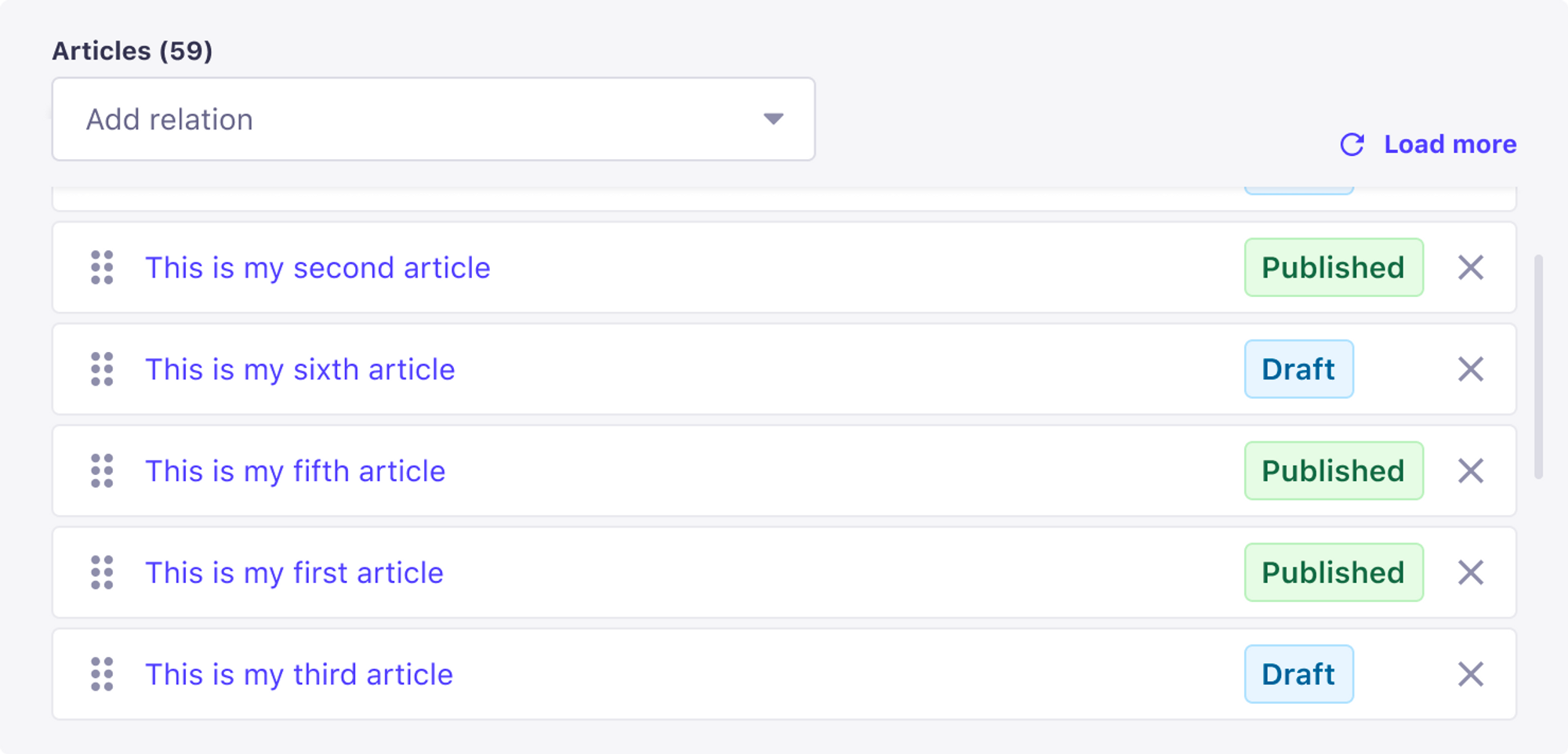This reordering feature is available in the Content Manager and the API.

## Code location​

`packages/core/database/lib/entity-manager/relations-orderer.js`

## How is the order stored in DB?​

• We store the order value of the relation in an `order` field.
• For bidirectional relations, we store the order value of the other side in an `inverse_order` field.

We store order values for all type of relations, except for:

• Polymorphic relations (too complicated to implement).
• One to one relations (as there is only one relation per pair)

### Many to many (Addresses <-> Categories)​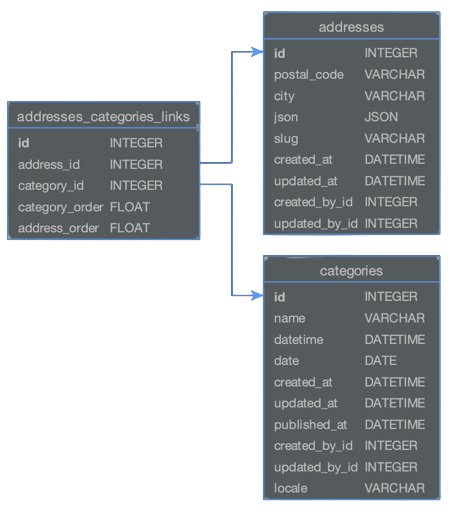• `category_order` is the order value of the categories relations in an address entity.
• `address_order` is the order value of the addresses relations in a category entity.

### One to one (Kitchensinks <-> Tags)​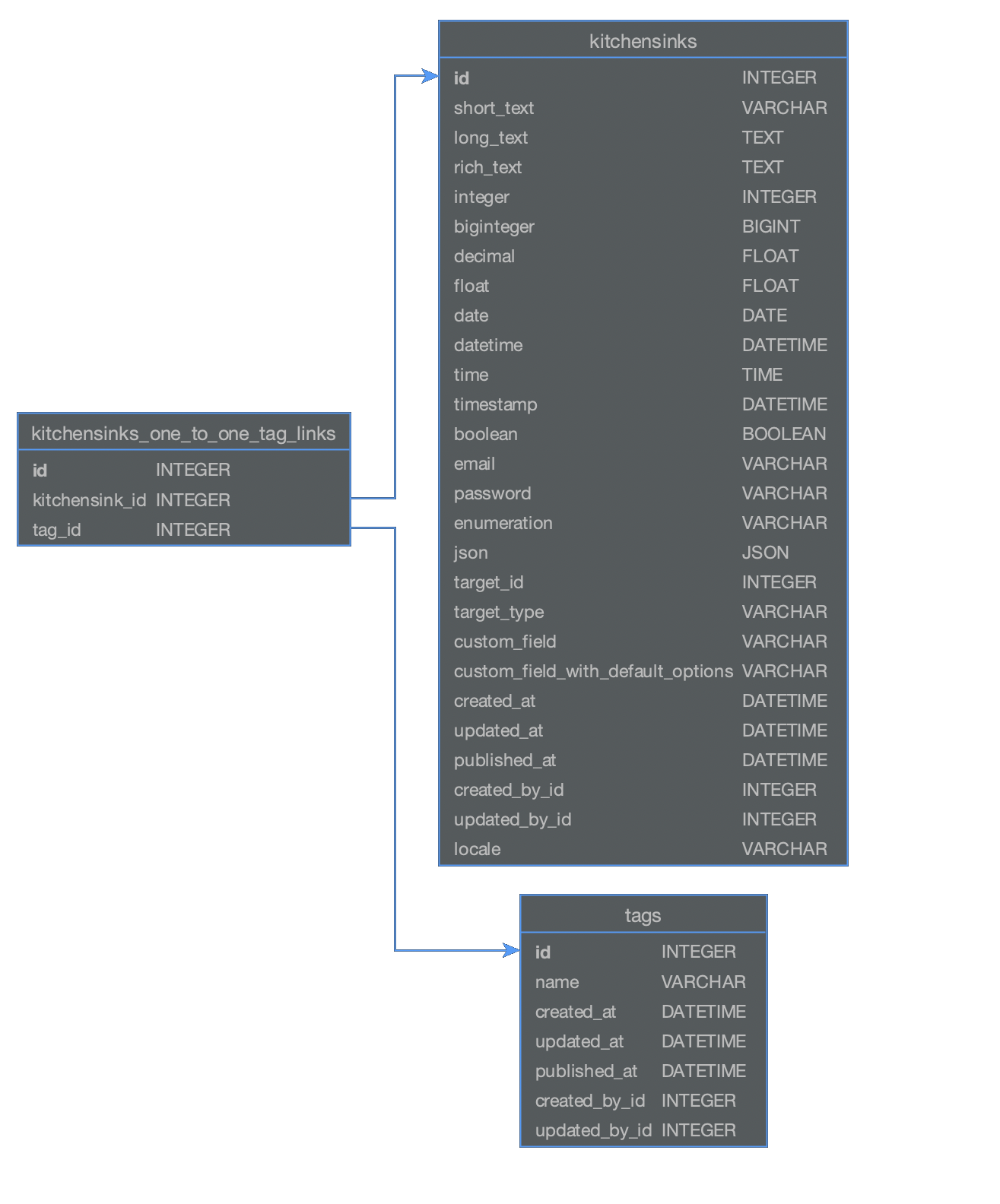• there is no `order` fields as there is only one relation per pair.

### One way relation (Restaurants <-> Categories)​

Where a restaurant has many categories: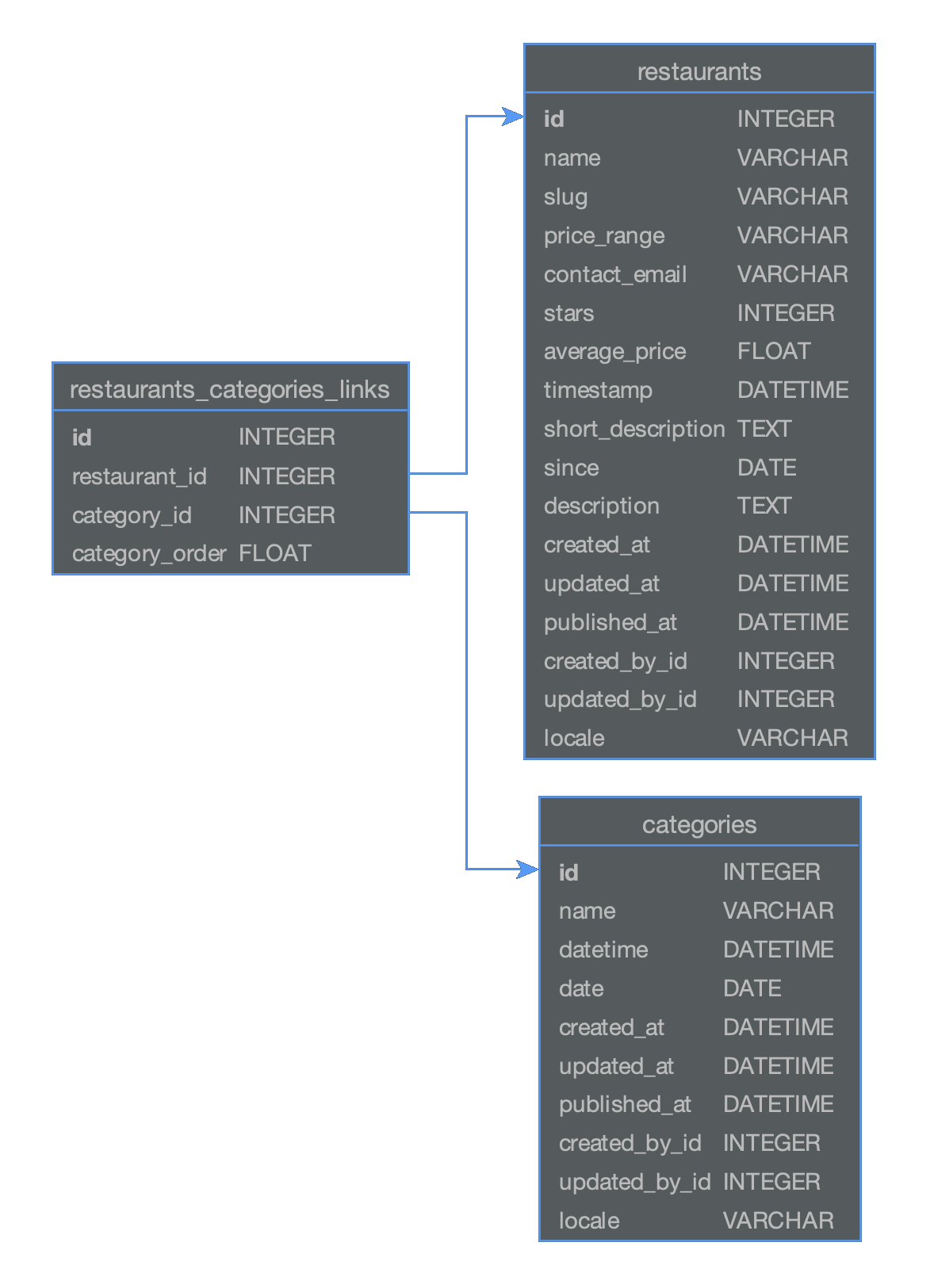• `category_order` is the order value of the categories relations in a restaurant entity.
• There is no `restaurant_order` as it is a one way relation.

## How to reorder relations in the DB layer​

See more on Strapi Docs

``  category: {    connect: [      { id: 6, position: { after: 1} },    // It should be after relation id=1      { id: 8, position: { end: true }},   // It should be at the end    ],    disconnect: [      { id: 4 }    ]  }``

## How does relations reordering work?​

We use fractional indexing. This means that we use decimal numbers to order the relations. See the following diagrams below for a more detailed understanding.

### Simple example​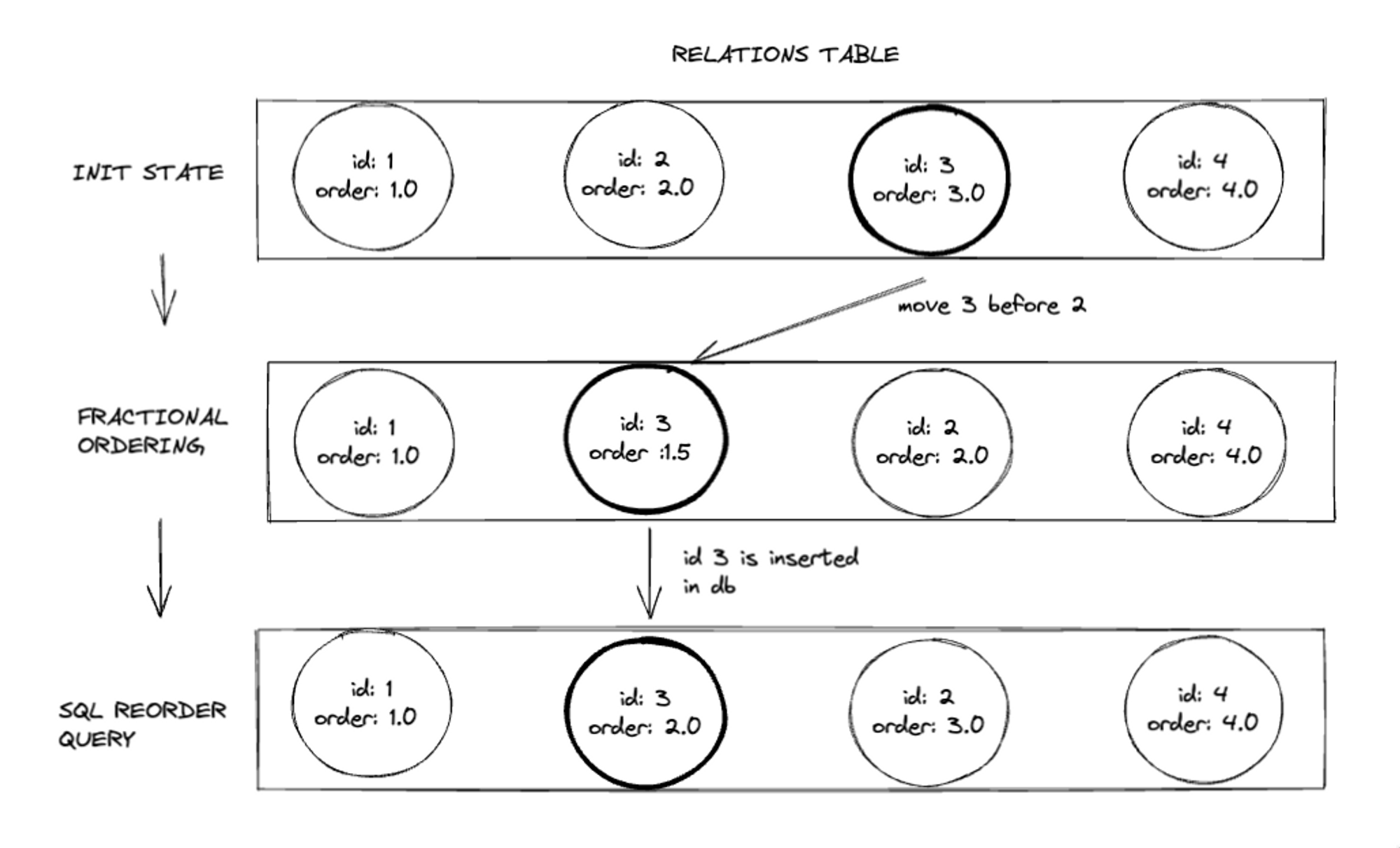### Complex example​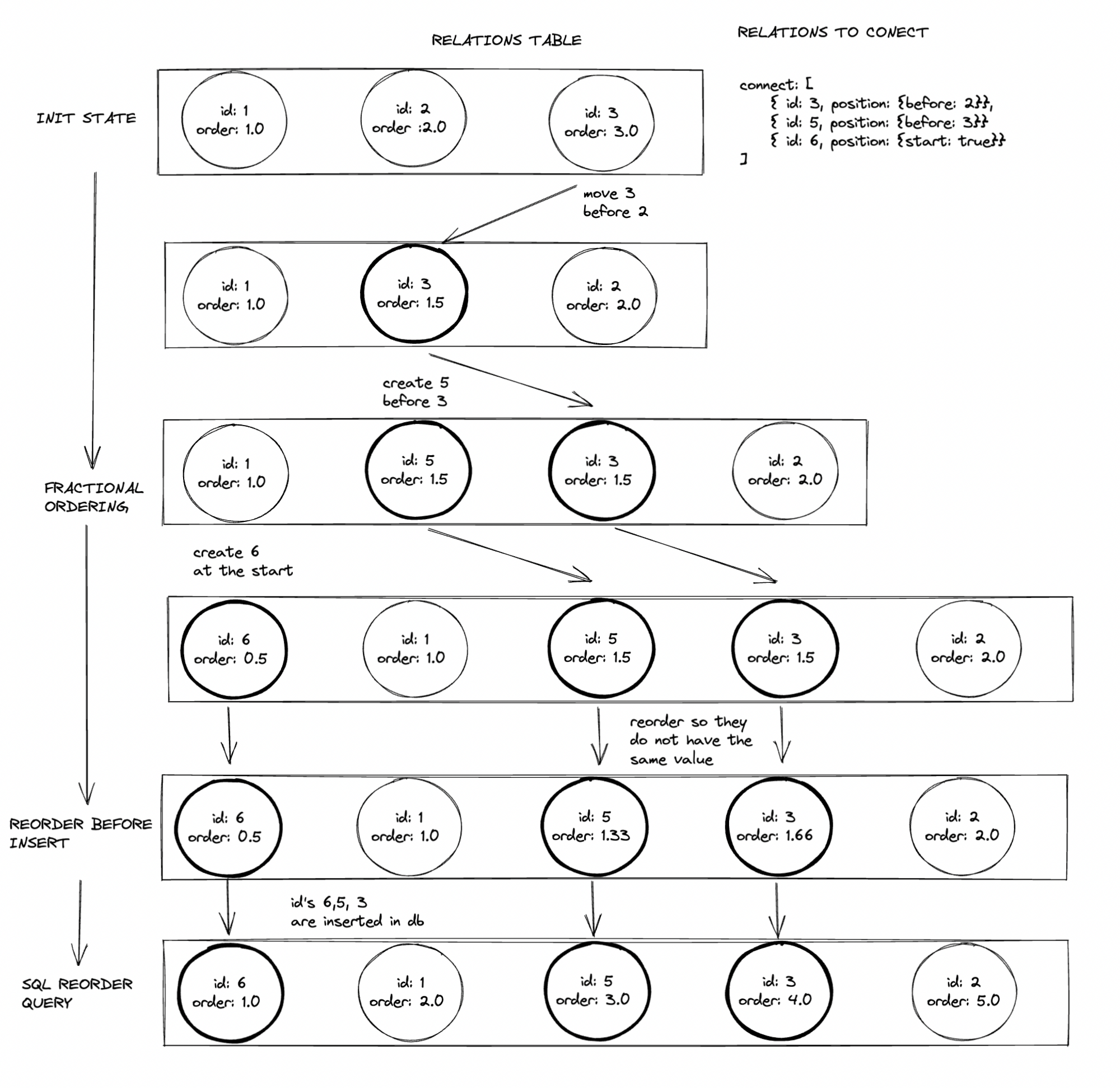### Algorithm steps​

From the `connect` array:

• For every element, load relations by id, from fields `after` or `before`.
• Start computing based on the `after` and `before` relations:
• Initialize with after/before relations (step 1). Let's call these ones init relations.
• Apply the updates from the `connect` array, sequentially.
• If the update is of type `before`:
• Place the element with the given `id` before the specified element in the list.
• If the specified element is an `init relation`, place the element in between that relation and the one before it.
• To determine the order value, order = beforeRelation.order - 0.5. This ensures the element is placed before the `before` relation and after the one before it.
• Else order = beforeRelation.order
• If the update is of type `after`:
• Place the element with the given `id` after the specified element in the list.
• If the specified element is an `init relation`, place the element in between that relation and the one after it.
• To determine the order value, order = beforeRelation.order + 0.5. This ensures the element is placed before the `after` relation and before the one after it.
• Else order = beforeRelation.order
• If the update is of type `end`:
• Place at the end
• If placing after an init relation: order = lastRelation.order + 0.5
• Else order = lastRelation.order
• If the update is of type `start`: -Place at the start
• order = 0.5
• `before/after`: If the id does not exist in the current array, throw an error
• If an id was already in this array, remove the previous one
• Grouping by the order value, and ignoring init relations
• Recalculate order values for each group, so there are no repeated numbers & they keep the same order.
• Example : [ {id: 5 , order: 1.5}, {id: 3, order: 1.5 } ][ {id: 5 , order: 1.33}, {id: 3, order: 1.66 } ]
• Insert values in the database
• Update database order based on their order position. (using ROW_NUMBER() clause)

From the disconnect array:

• Delete the relations from the database.
• Reorder the remaining elements in the database based on their position, using ROW_NUMBER() clause.